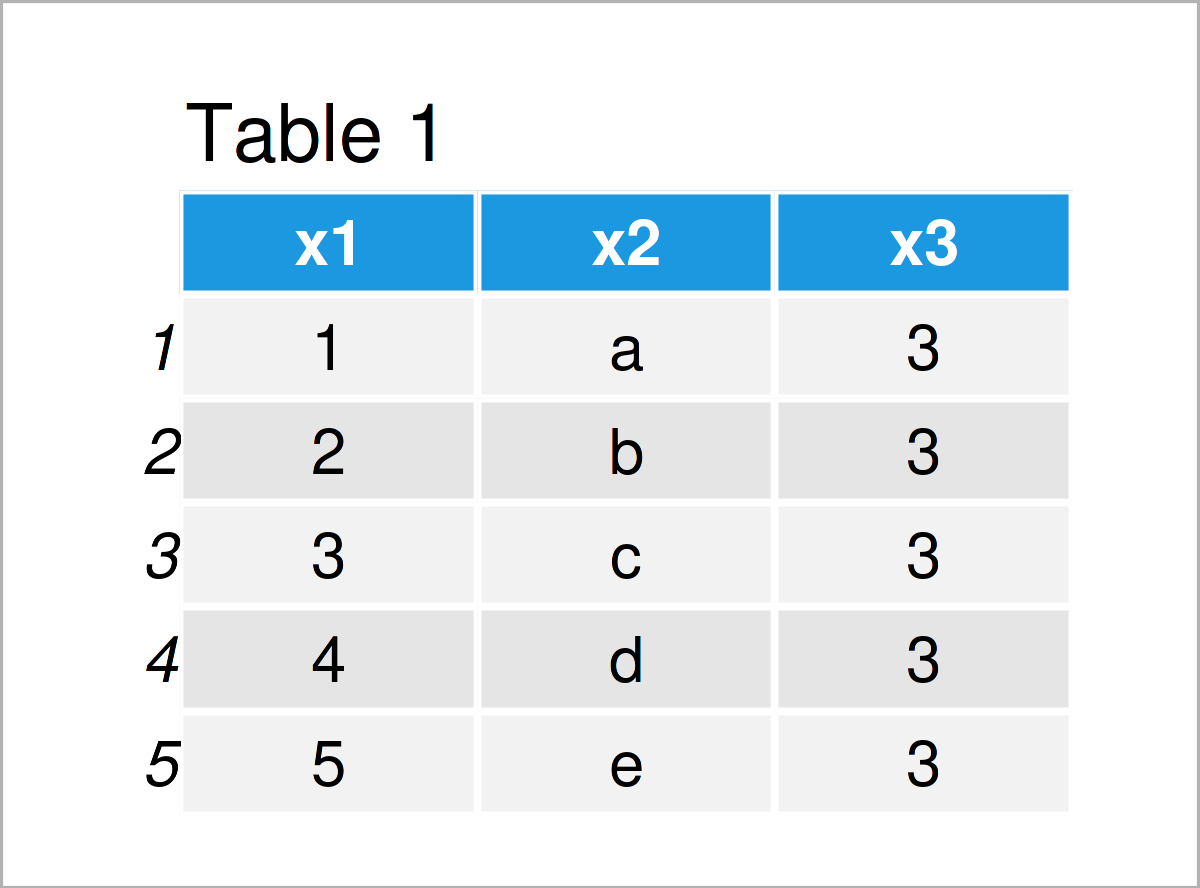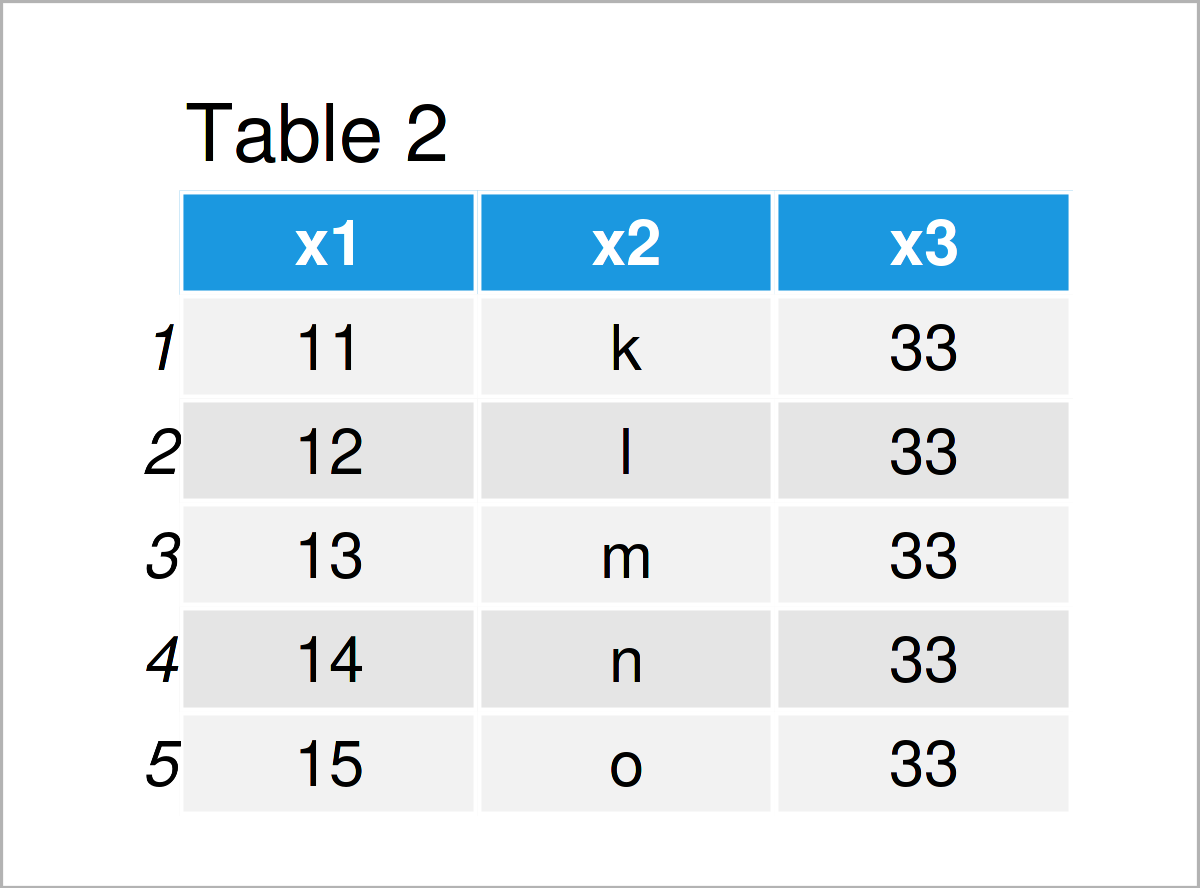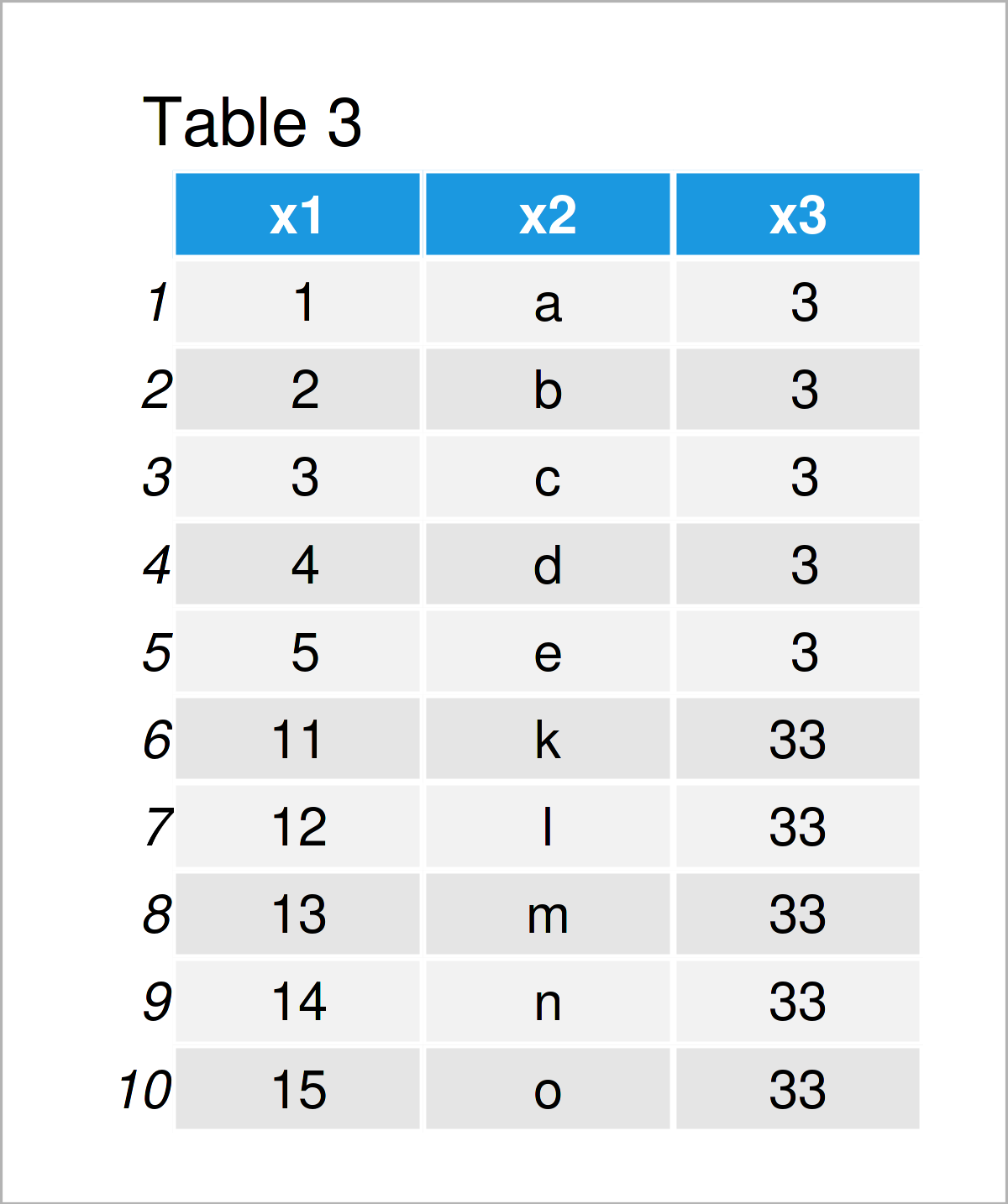# Append data.table to Another in R – Concatenate Two Tables (Example)

This tutorial explains how to append two data.tables vertically in the R programming language.

The content is structured as follows:

Let’s get started…

## Example Data & Packages

First, we need to install and load the data.table package:

```install.packages("data.table")                  # Install & load data.table
library("data.table")```

The following data.tables are used as a basis for this R tutorial:

```data1 <- data.table(x1 = 1:5,                   # Create first data.table
x2 = letters[1:5],
x3 = 3)
data1                                           # Print first data.table``````data2 <- data.table(x1 = 11:15,                 # Create second data.table
x2 = letters[11:15],
x3 = 33)
data2                                           # Print second data.table```In Tables 1 and 2 it is shown that we have created two new data.tables using the previous R code. Both of these data sets contain the same columns, i.e. x1, x2, and x3.

## Example: Concatenate List of Two data.tables Using rbindlist() Function

This example explains how to join multiple data.tables vertically.

For this task, we are using the rbindlist function of the data.table package in combination with the list function.

```data_concat <- rbindlist(list(data1, data2))    # Rbind data.tables
data_concat                                     # Print combined data.table```The output of the previous R syntax is illustrated in Table 3: We have created a data.table union of our two input data sets where the first data.table is stacked on top of the second data.table.

If you want to learn more on why he rbindlist function might have advantages compared to other binding functions such as rbind of Base R or bind_rows of the dplyr package, please have a look here.

## Video & Further Resources

In case you need further information on the R code of this article, you may have a look at the following video that I have published on my YouTube channel. I’m explaining the R code of this tutorial in the video:

Please accept YouTube cookies to play this video. By accepting you will be accessing content from YouTube, a service provided by an external third party.If you accept this notice, your choice will be saved and the page will refresh.

Furthermore, you might want to have a look at the other tutorials on my website. You can find a selection of articles on similar topics such as data.table and merging below.

In this article, I have explained how to combine two data.tables in R. Let me know in the comments below, if you have further questions or comments.

Subscribe to the Statistics Globe Newsletter